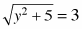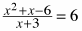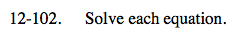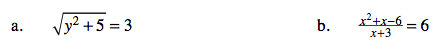### Home > CAAC > Chapter 12 > Lesson 12.4.4 > Problem12-102

12-102.
1. Solve each equation. Homework Help ✎

1.2.Square both sides of the equation.

Solve for y.

Multiply both sides of the equation by ( x + 3 ).

Set the equation equal to zero.

Solve for x.